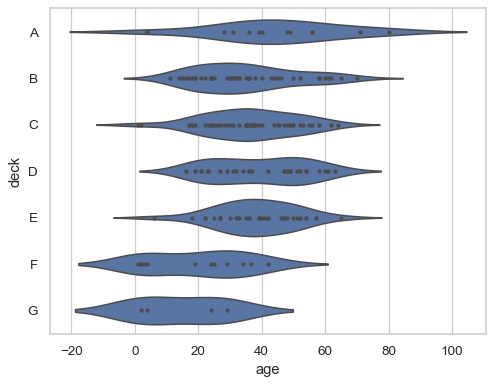# seaborn.violinplot#

seaborn.violinplot(data=None, *, x=None, y=None, hue=None, order=None, hue_order=None, bw='scott', cut=2, scale='area', scale_hue=True, gridsize=100, width=0.8, inner='box', split=False, dodge=True, orient=None, linewidth=None, color=None, palette=None, saturation=0.75, ax=None, **kwargs)#

Draw a combination of boxplot and kernel density estimate.

A violin plot plays a similar role as a box and whisker plot. It shows the distribution of quantitative data across several levels of one (or more) categorical variables such that those distributions can be compared. Unlike a box plot, in which all of the plot components correspond to actual datapoints, the violin plot features a kernel density estimation of the underlying distribution.

This can be an effective and attractive way to show multiple distributions of data at once, but keep in mind that the estimation procedure is influenced by the sample size, and violins for relatively small samples might look misleadingly smooth.

Note

This function always treats one of the variables as categorical and draws data at ordinal positions (0, 1, … n) on the relevant axis, even when the data has a numeric or date type.

Parameters:
dataDataFrame, array, or list of arrays, optional

Dataset for plotting. If `x` and `y` are absent, this is interpreted as wide-form. Otherwise it is expected to be long-form.

x, y, huenames of variables in `data` or vector data, optional

Inputs for plotting long-form data. See examples for interpretation.

order, hue_orderlists of strings, optional

Order to plot the categorical levels in; otherwise the levels are inferred from the data objects.

bw{‘scott’, ‘silverman’, float}, optional

Either the name of a reference rule or the scale factor to use when computing the kernel bandwidth. The actual kernel size will be determined by multiplying the scale factor by the standard deviation of the data within each bin.

cutfloat, optional

Distance, in units of bandwidth size, to extend the density past the extreme datapoints. Set to 0 to limit the violin range within the range of the observed data (i.e., to have the same effect as `trim=True` in `ggplot`.

scale{“area”, “count”, “width”}, optional

The method used to scale the width of each violin. If `area`, each violin will have the same area. If `count`, the width of the violins will be scaled by the number of observations in that bin. If `width`, each violin will have the same width.

scale_huebool, optional

When nesting violins using a `hue` variable, this parameter determines whether the scaling is computed within each level of the major grouping variable (`scale_hue=True`) or across all the violins on the plot (`scale_hue=False`).

gridsizeint, optional

Number of points in the discrete grid used to compute the kernel density estimate.

widthfloat, optional

Width of a full element when not using hue nesting, or width of all the elements for one level of the major grouping variable.

inner{“box”, “quartile”, “point”, “stick”, None}, optional

Representation of the datapoints in the violin interior. If `box`, draw a miniature boxplot. If `quartiles`, draw the quartiles of the distribution. If `point` or `stick`, show each underlying datapoint. Using `None` will draw unadorned violins.

splitbool, optional

When using hue nesting with a variable that takes two levels, setting `split` to True will draw half of a violin for each level. This can make it easier to directly compare the distributions.

dodgebool, optional

When hue nesting is used, whether elements should be shifted along the categorical axis.

orient“v” | “h”, optional

Orientation of the plot (vertical or horizontal). This is usually inferred based on the type of the input variables, but it can be used to resolve ambiguity when both `x` and `y` are numeric or when plotting wide-form data.

linewidthfloat, optional

Width of the gray lines that frame the plot elements.

colormatplotlib color, optional

Single color for the elements in the plot.

palettepalette name, list, or dict

Colors to use for the different levels of the `hue` variable. Should be something that can be interpreted by `color_palette()`, or a dictionary mapping hue levels to matplotlib colors.

saturationfloat, optional

Proportion of the original saturation to draw colors at. Large patches often look better with slightly desaturated colors, but set this to `1` if you want the plot colors to perfectly match the input color.

axmatplotlib Axes, optional

Axes object to draw the plot onto, otherwise uses the current Axes.

Returns:
axmatplotlib Axes

Returns the Axes object with the plot drawn onto it.

`boxplot`

A traditional box-and-whisker plot with a similar API.

`stripplot`

A scatterplot where one variable is categorical. Can be used in conjunction with other plots to show each observation.

`swarmplot`

A categorical scatterplot where the points do not overlap. Can be used with other plots to show each observation.

`catplot`

Combine a categorical plot with a `FacetGrid`.

Examples

Draw a single horizontal boxplot, assigning the data directly to the coordinate variable:

```df = sns.load_dataset("titanic")
sns.violinplot(x=df["age"])
```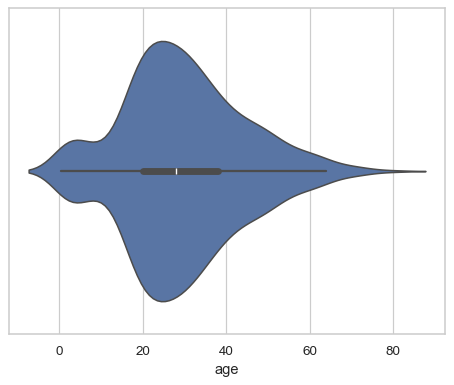Group by a categorical variable, referencing columns in a dataframe:

```sns.violinplot(data=df, x="age", y="class")
```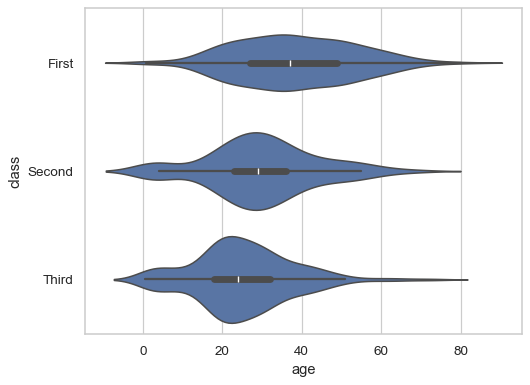Draw vertical violins, grouped by two variables:

```sns.violinplot(data=df, x="class", y="age", hue="alive")
```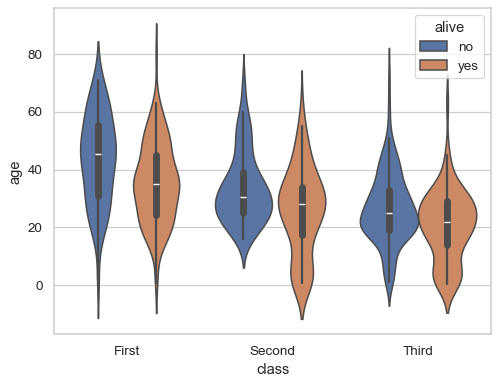Draw split violins to take up less space:

```sns.violinplot(data=df, x="deck", y="age", hue="alive", split=True)
```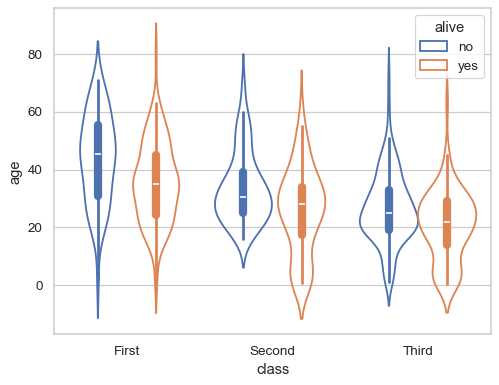Prevent the density from smoothing beyond the limits of the data:

```sns.violinplot(data=df, x="age", y="alive", cut=0)
```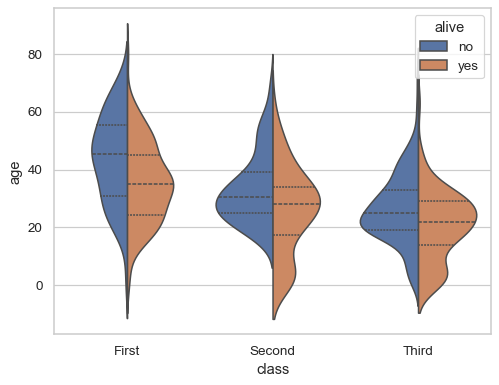Use a narrower bandwidth to reduce the amount of smoothing:

```sns.violinplot(data=df, x="age", y="alive", bw=.15)
```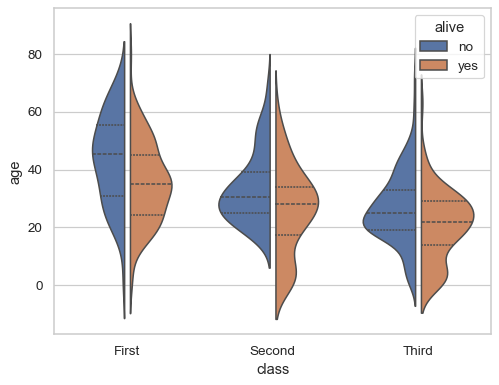Represent every observation inside the distribution

```sns.violinplot(data=df, x="age", y="embark_town", inner="stick")
```Use a different scaling rule for normalizing the density:

```sns.violinplot(data=df, x="age", y="embark_town", scale="count")
```